#### How to Make Correlation Graph in Excel

Correlation helps to identify the pattern of change between two variables. It has two properties – strength and direction. It is a common statistics concept which can be easily represented in Excel using a scatter chart and a trend line.

## What is Correlation Chart?

Correlation Chart is a type of graph or mathematical scheme that uses Cartesian coordinates for display values typically of two variables for a data set. The data is displayed as a collection of points, each with the value of one position variable on the horizontal axis and the value of a second position variable on the vertical axis.

## Direction of Correlation

• Positive:

If the correlation coefficient is positive, it shows a direct proportional relation between the variables and an upward slope of the correlation graph.

If variable 1 increases, variable 2 will also increase – and vice versa.

• Negative:

If the correlation coefficient is negative, it shows an inverse proportional relation between the variables and a downward slope of the correlation graph.

If variable 1 increases, variable 2 will also decrease – and vice versa.

## Create Correlation Chart in Excel

Step 1: Create the correlation dataset. The independent variable should be in the left-hand side (X-axis) and the dependent variable should be in right-hand side (Y-axis).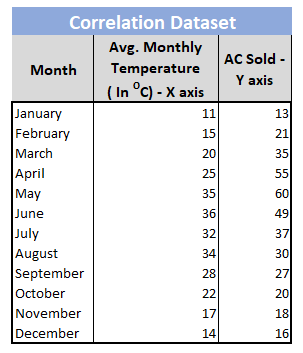Step 2: Select the column for X-axis and Y-axis.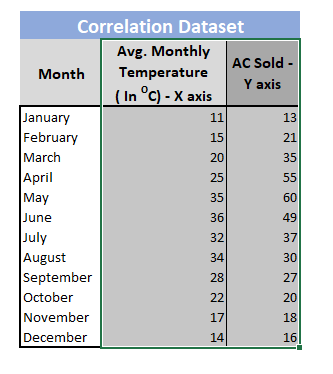Step 3: Insert -> Charts -> Scatter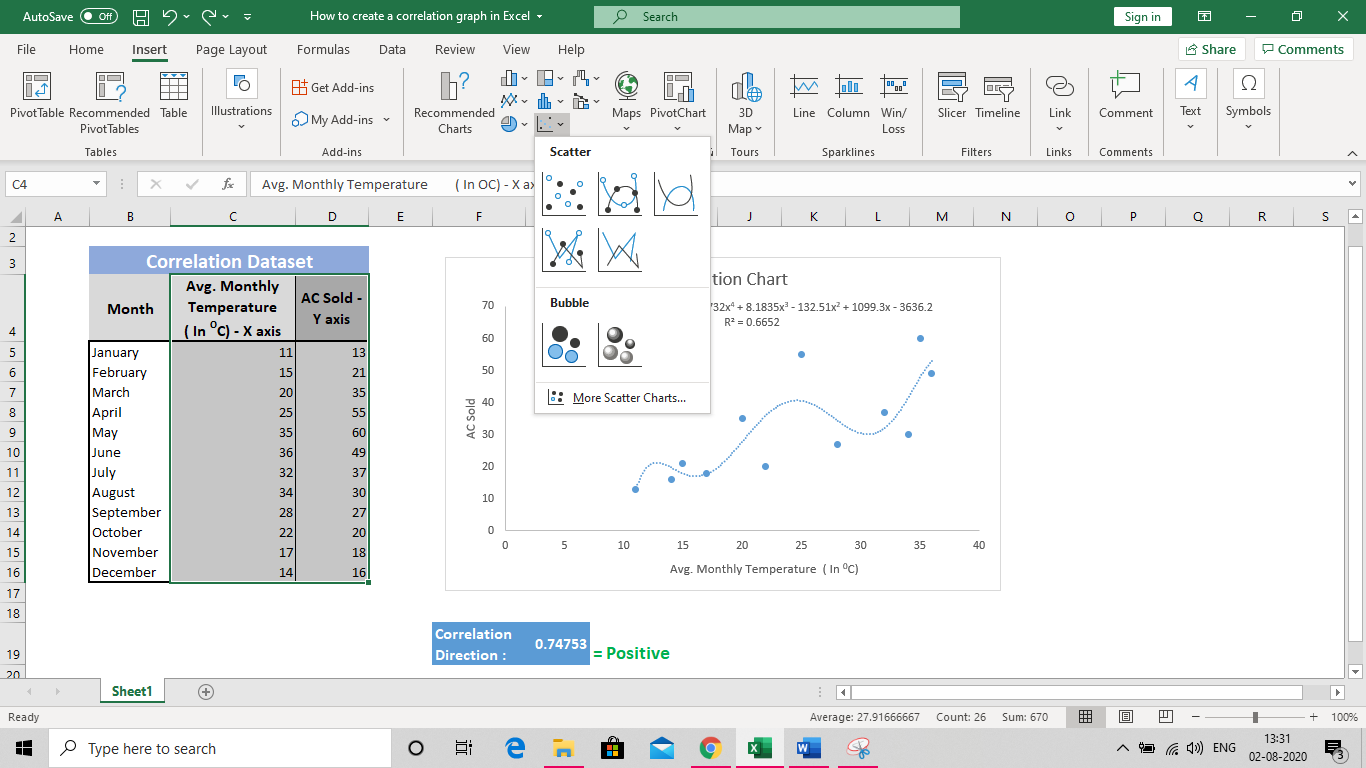Step 4: Name X-axis and Y-axis. Name the chart.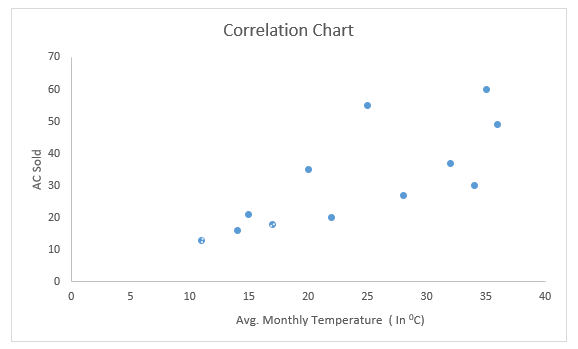Step 5: Right Click on any datapoint. Add trendline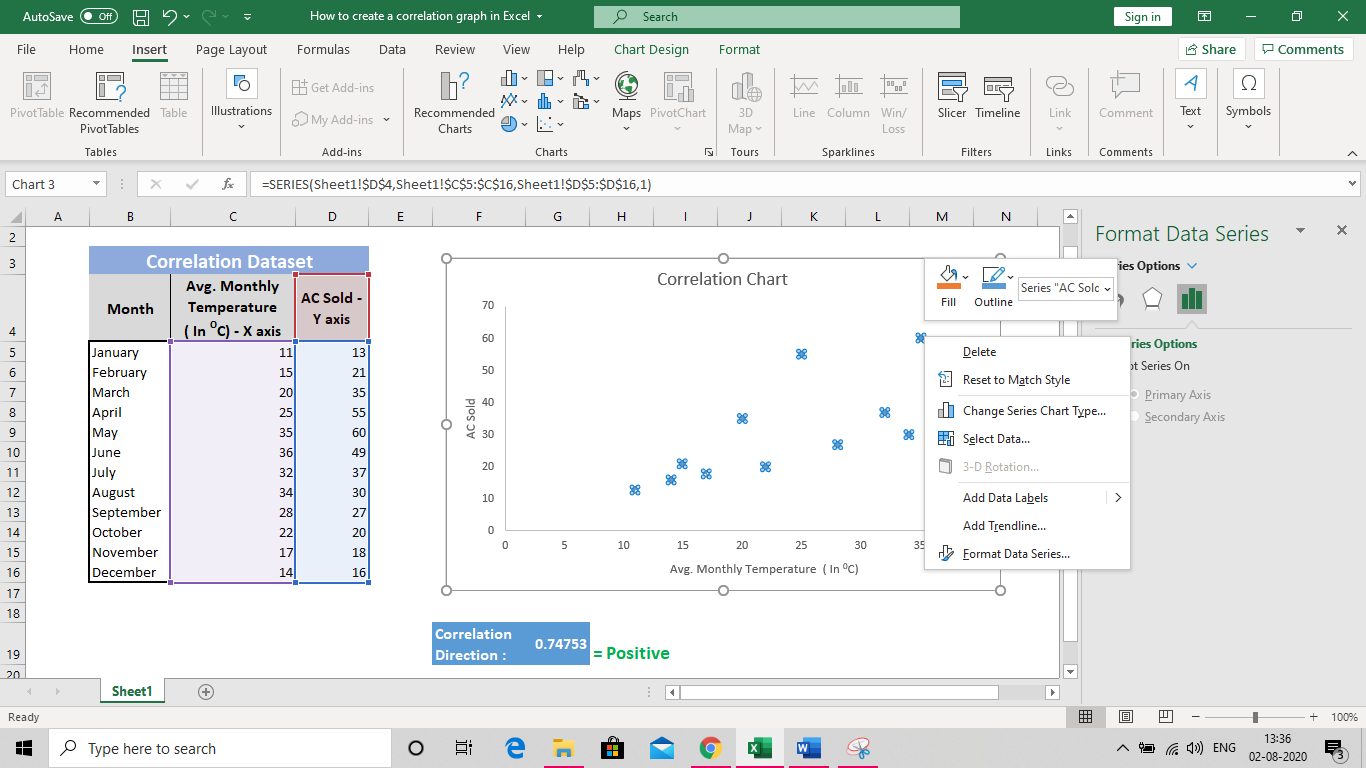Step 6: Select the most suitable trendline. Check the following boxes as shown below.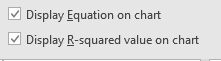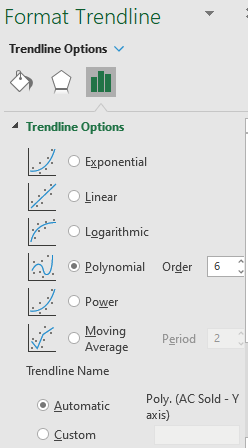Step 8: The final Correlation Graph is created.

## Generate Correlation Direction to Find Positive/Negative Relation

Syntax: = Correl(array1, array2)

Where:

Array1 is the first data range of values.

Array2 is the second data range of values.

Value in Example Above: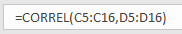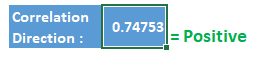Errors:

• #N/A: If the two arrays are not same in length
• #DIV/0!: If either of the array is blank or standard deviation of the numbers is 0.

## Interpreting Scatter Plots

Scatter plots visually represent data points for two variables on a graph. The pattern of these points helps in understanding correlations:

• Positive correlation: Points tend to form an upward-sloping line.
• Negative correlation: Points tend to form a downward-sloping line.
• No correlation: Points appear scattered with no apparent pattern.

## Real-World Applications

Correlation and scatter plots find applications in various fields, such as finance (stock price vs. company earnings), healthcare (relationship between age and blood pressure), and marketing (advertising spend vs. sales).